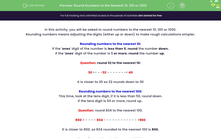# Round Numbers to the Nearest 10, 100 or 1,000

In this worksheet, students will be asked to round numbers accurately to the nearest 10, 100 or 1,000.Key stage:  KS 2

Curriculum topic:   Number: Number and Place Value

Curriculum subtopic:   Round Numbers for Accuracy

Popular topics:   Rounding worksheets, Estimation worksheets

Difficulty level:#### Worksheet Overview

In this activity, you will be asked to round numbers to the nearest 10, 100 or 1,000.

Rounding numbers means adjusting the digits (either up or down) to make rough calculations simpler.

Rounding numbers to the nearest 10:

If the 'ones' digit of the number is less than 5, round the number down.

If the 'ones' digit of the number is 5 or more, round the number up.

Question: round 32 to the nearest 10:

30 <- - -32 - - - - - - -> 40

It is closer to 30 so 32 rounds down to 30

Rounding numbers to the nearest 100:

This time, look at the tens digit, if it is less than 50, round down.

If the tens digit is 50 or more, round up.

Question: round 834 to the nearest 100.

800 < - - - - 834 - - - - - - - - - - - - >900

It is closer to 800, so 834 rounded to the nearest 100 is 800.

Rounding numbers to the nearest 1,000:

When rounding to the nearest 1,000, count in 1,000s either side of your number and choose the nearest.

Question: round 2,839 to the nearest 1,000

2,000 <- - - - - - - - - - - - - - - -2,839 - - - - > 3,000

It's closer to 3,000, so 2,839 rounded to the nearest 1,000 is 3,000.

That's a lot to remember so feel free to check back at this introduction at any point by clicking on the red help button that will appear on the screen when you start the questions.### What is EdPlace?

We're your National Curriculum aligned online education content provider helping each child succeed in English, maths and science from year 1 to GCSE. With an EdPlace account you’ll be able to track and measure progress, helping each child achieve their best. We build confidence and attainment by personalising each child’s learning at a level that suits them.

Get started